# Flow in a network

(Redirected from Max-flow-min-cut theorem)

A function which assigns certain numbers to the arcs of a given network graph (directed graph). Each number is interpreted as the intensity of the flow along the given arc. Flows in a network form a convenient model in the studies of a number of problems in transportation, communications, and other areas related to the traffic of goods, the transmission of information, etc. Many problems concerning flows are problems in linear programming and can be solved by the general methods of this theory. However, the majority of problems concerning flows admit an efficient solution by methods of graph theory.

To each arc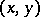of a networklet a non-negative real number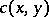be assigned — the transmission capacity of the arc. The flow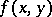is said to be a stationary flow of valuefrom a vertexto a vertexsatisfying the restrictions on transmission capacities of the arcs if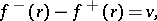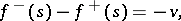Hereis the flow leaving the vertexand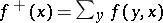is the flow entering the vertex. In the problem of maximum flow (or max-flow problem) between two vertices, one has to construct a stationary flow from a vertexto a vertexof maximum possible value. There are efficient algorithms to solve this problem. Letbe a subset of vertices ofsuch that,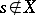. Then the set of arcssuch that,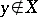is called a cut. The transmission capacity of a cut is the value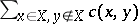. The following theorem on maximum flow and minimum cut (or max-flow-min-cut theorem) holds: The maximum value of a flow is equal to the minimum transmission capacity of the cuts. In applications one often uses the integrality theorem: If the transmission capacity of each arc is an integer, then there exists an integral maximum (stationary) flow.

A number of problems can be reduced to the problem of maximum flow between two vertices: the problem of maximum flow in a network with several sources and sinks; the problem of maximum flow in a network with non-negative lower and upper bounds on the flow along an arc; the problem of maximum flow in undirected and mixed networks; the problem of maximum flow in a network with given transmission capacities of arcs and vertices; etc.

The max-flow-min-cut theorem has revealed the common basis of a number of results obtained before in graph theory and combinatorial analysis. It turned out that the following theorems can be obtained as corollaries of this theorem: the maximum matching theorem for a bipartite graph (cf. Graph, bipartite), the theorem on distinct representatives, theorems on the-connectivity of graphs (see Graph, connectivity of a), the theorem on the covering of a partially ordered set by a minimum number of chains, etc. Reduction of different problems to the problem of maximum flow is an important method in graph theory and combinatorial analysis.

In a number of problems on flows in a network a number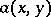corresponds to each arc— the cost of transportation of a unit of goods along— and one has to find a flow which, subject to certain constraints, minimizes the total cost of the flow. The problem of a flow of minimum cost (or min-cost-flow problem) consists in finding a stationary flow from a vertexto a vertexsubject to constraints on the transmission capacities of the arcs and such that its absolute value is equal to a given numberand its cost is minimal.

In transportation problems the network is a bipartite graph. Vertices of one partare interpreted as departure points for some goods, and the vertices of the other part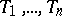as destination points. Each departure point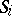has a certain supply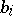and each destination point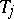has a certain demand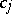. Let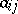, the cost of transportation per unit of goods fromto, be given. The problem consists in finding a flow of minimum cost subject to the demands at all destination points.

One can also consider multi-product flows and flows changing in time.

How to Cite This Entry:
Max-flow-min-cut theorem. Encyclopedia of Mathematics. URL: http://encyclopediaofmath.org/index.php?title=Max-flow-min-cut_theorem&oldid=42251